Like   Pin

## Thursday, October 24, 2019

 table div table+table+table div table{width:100%;padding:0}table div table+table+table div table img{width:96.23%;padding:0;float:none}table div table+table+table div table td{width:100%;padding:0 1.88% 18px}/* styles */# Sunday's Service - October 27, 2019

 /* styles */ We welcome back John Muckridge, President & CEO of the Rescue Mission of Mahoning Valley, to lead us in Worship this week. The featured Scripture comes from: Matthew 27:51-61 for John's message: Bearing Witness to Jesus’ Death.
 /* styles */ ~~~

# CG NEWS & EVENTS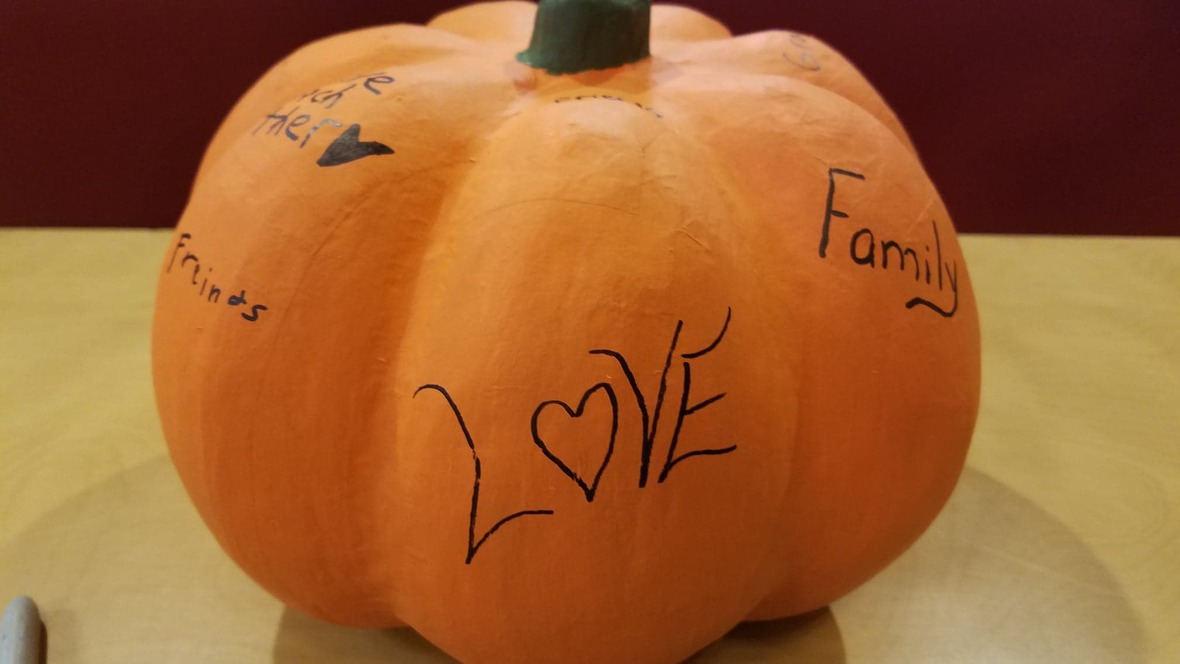## Fall Harvest & CG Birthday Celebration

Thanks to all who helped us celebrate! We had a
fantastic day of fun and fellowship!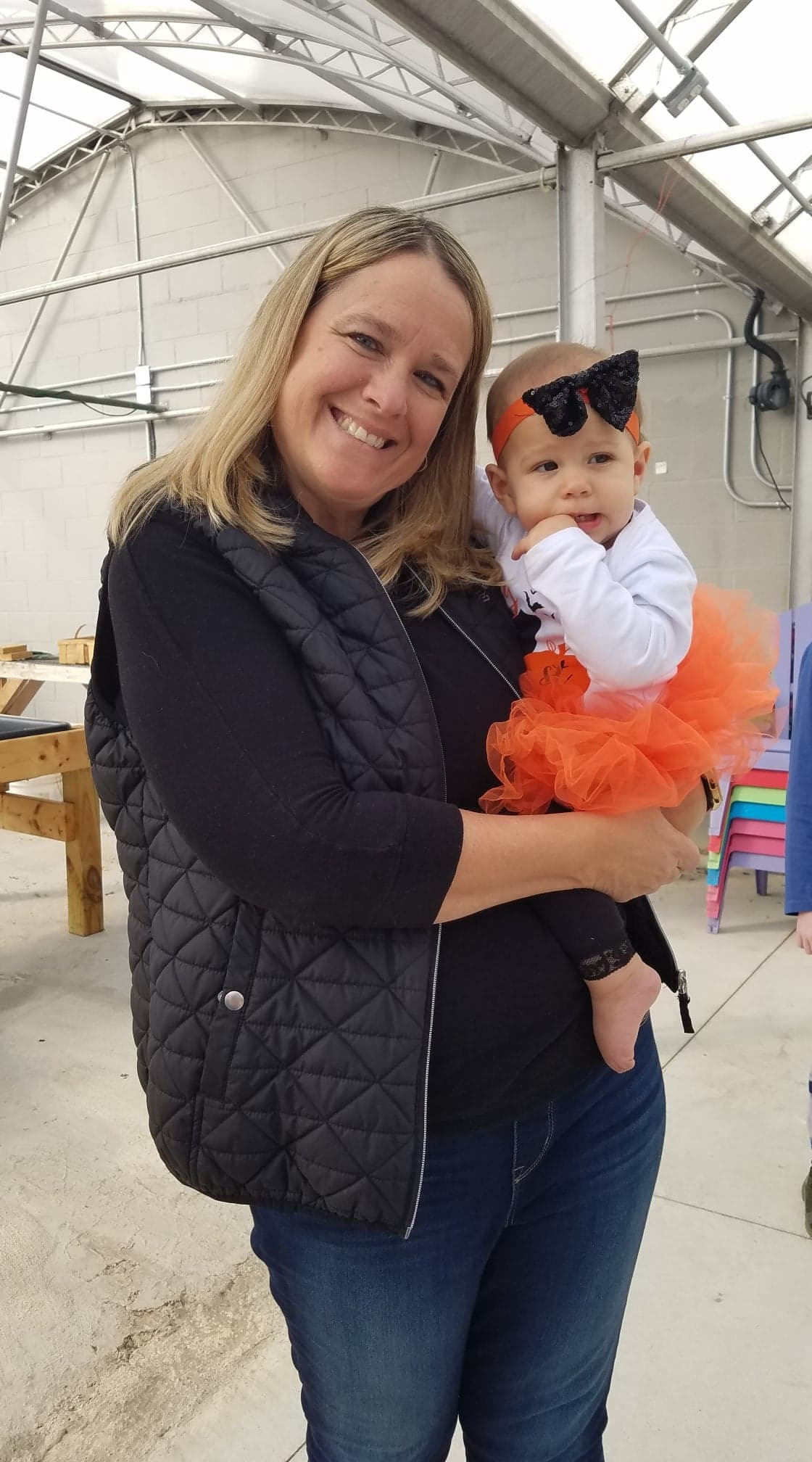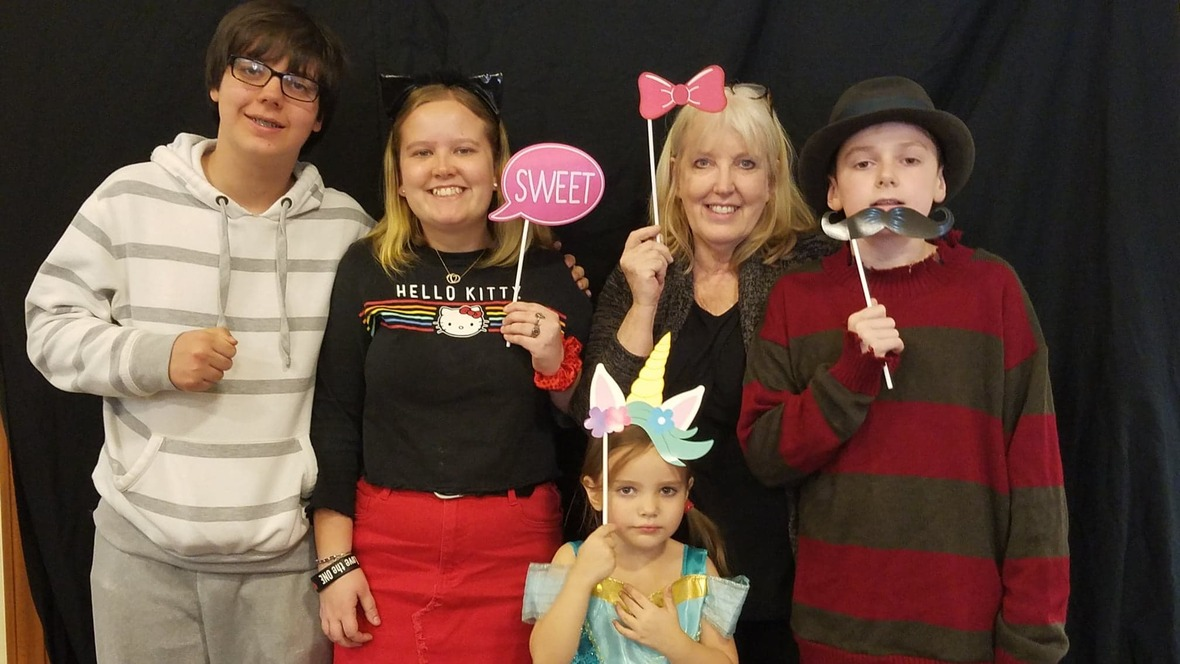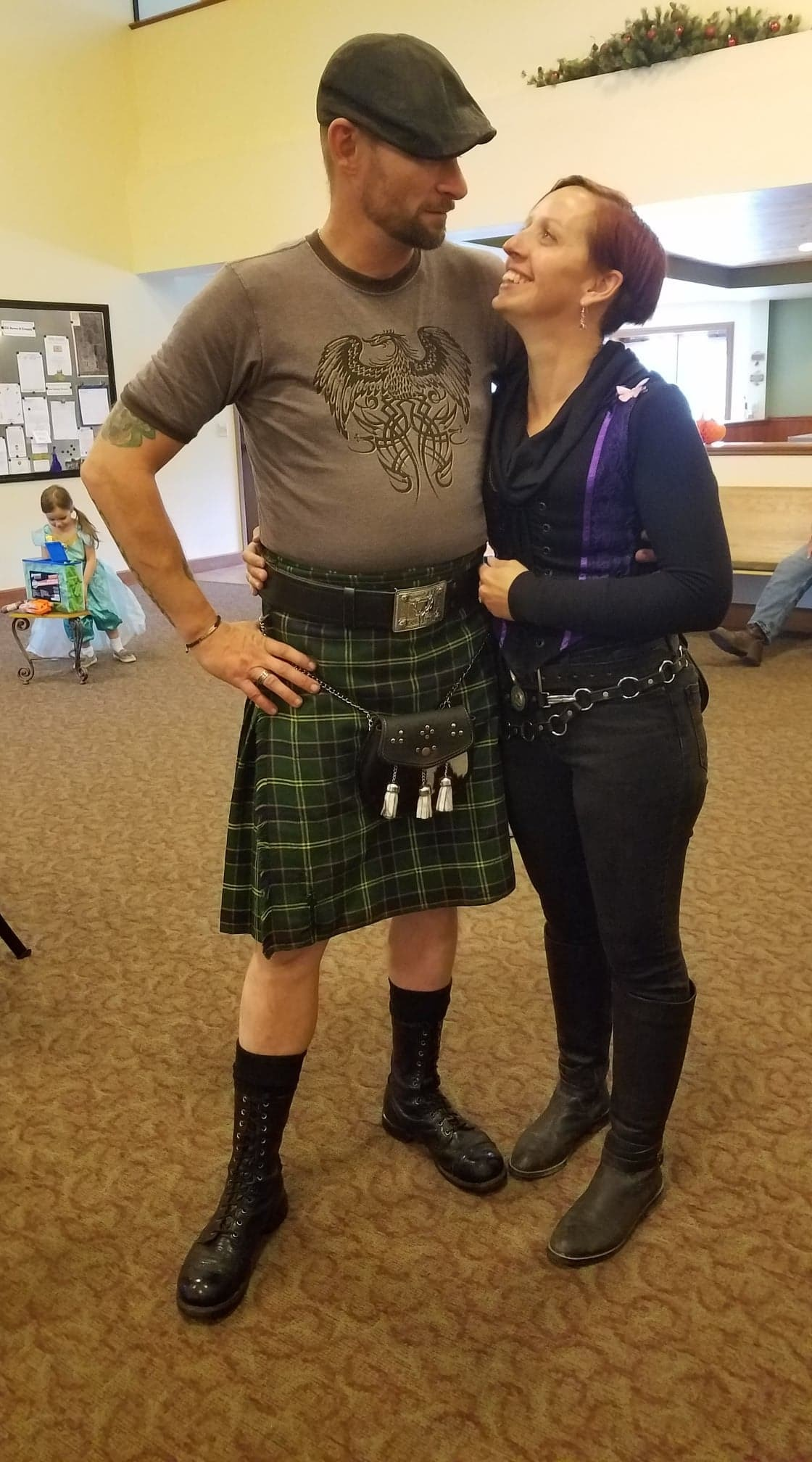## Bible Studies

Sunday Morning Class: Next meeting to be announced
We have finished the Book of Romans and will be taking a short break as Pat preps for the next class on the Book of Psalms.

 /* styles */ Wednesday Morning Ladies Study: 9:30am Best Friends with God: Falling in Love with the God Who Loves You by Christy Bower. You are welcome to join in studies at any time!

## Garden & Nursery News

Jen Shannon will be in the Garden this week with a lesson about Peace. Activity pages for kids on the table by Sanctuary doors. Children 3 & younger are invited to the Nursery for Bible story time.

Want to volunteer in the Garden and/or with the Sprouts and Nursery age kids?
We would love to have you jump on board! Talk to Jen L. for more information.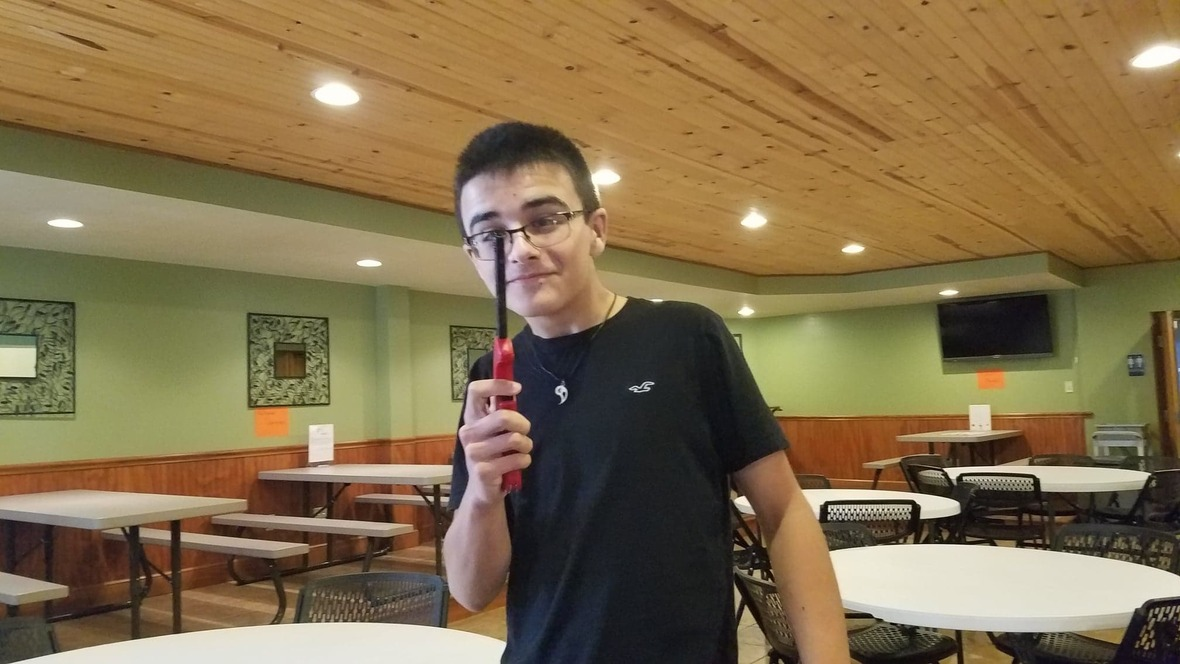## Youth Group

For youth in 5th grade & up

Back to our normal YG schedule this week!
Snack: Audrey & Nathan Shannon

We invite all the youth to join us for fun, fellowship, and learning this year,
most Sunday evenings from 6-7:30pm.

## Flu Shot Sunday

Didn't get your shot last Sunday? Pat will be manning his
table in the Cafe again this Sunday!

Great big thanks to Dr. Pat for once again providing flu shots to our church family! While not perfect, the seasonal flu shot protects against the three or four influenza viruses that research indicates will be most common during the season and can reduce the severity of a bout of the flu. Get protected this season!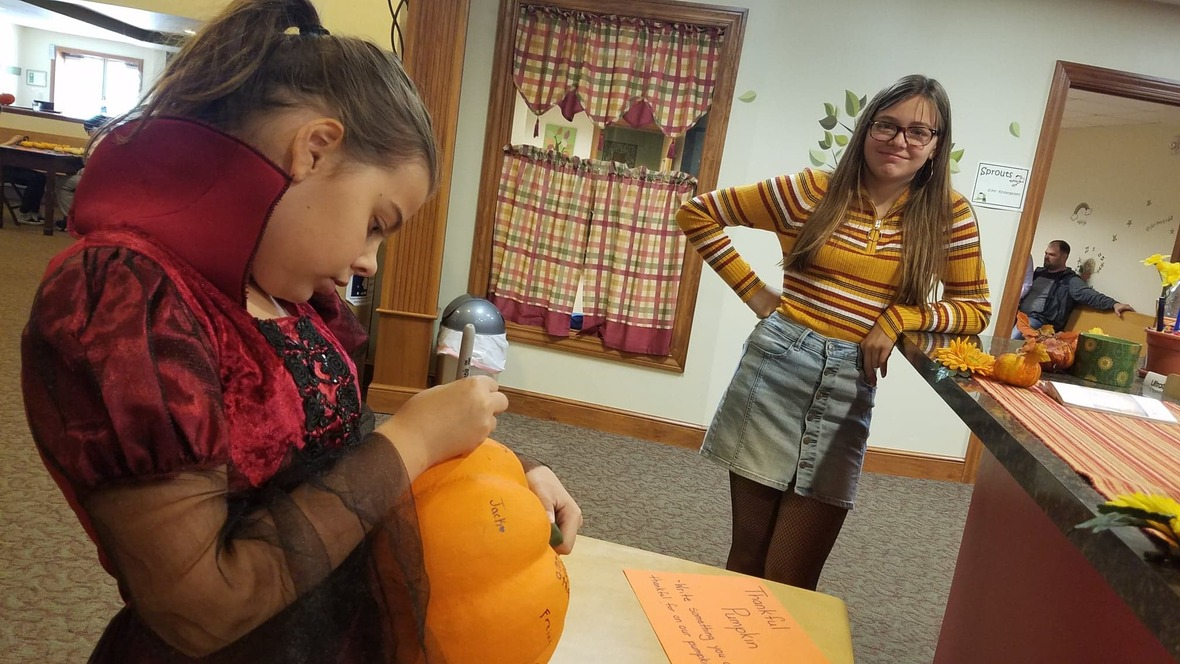## Thankful Pumpkin

Stop by the front desk and check out our "Thankful Pumpkin." Take a moment to add something for which you are thankful!

We are thankful for YOU!

 table div table+table+table+table+table+table+table+table+table+table+table+table+table+table+table+table+table div table{width:100%;padding:0}table div table+table+table+table+table+table+table+table+table+table+table+table+table+table+table+table+table div table img{width:96.23%;padding:0;float:none}table div table+table+table+table+table+table+table+table+table+table+table+table+table+table+table+table+table div table td{width:100%;padding:0 1.88% 18px}/* styles */# UPCOMING AT CG## November Speakers

3rd - Mick Naples
10th - Mick Naples
17th - Rev. David Hill
24th - Rev. David Hill

 table div table+table+table+table+table+table+table+table+table+table+table+table+table+table+table+table+table+table+table+table div table{width:100%;padding:0}table div table+table+table+table+table+table+table+table+table+table+table+table+table+table+table+table+table+table+table+table div table img{width:96.23%;padding:0;float:none}table div table+table+table+table+table+table+table+table+table+table+table+table+table+table+table+table+table+table+table+table div table td{width:100%;padding:0 1.88% 18px}/* styles *//* styles */ www.goodnessgrows4all.org Our Mission Statement: To cultivate personal, social, and community growth through gardening, education, and opportunity for people of all abilities. ~~~

# NEWS & EVENTS

## Fall at GG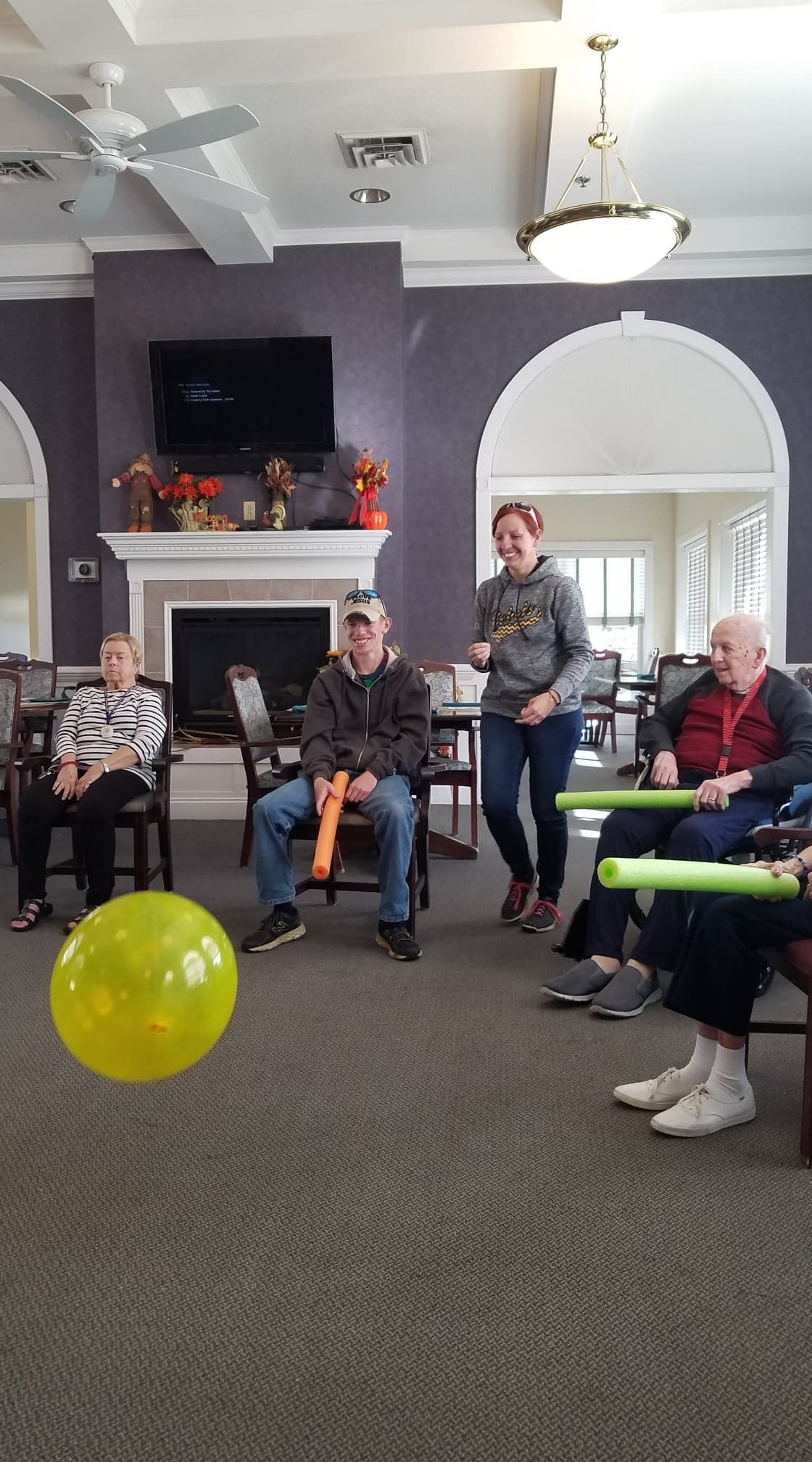We spent Monday morning at Whispering Pines, exercising with the residents. Everyone had a great time. Then we went shopping in Salem for gardening and painting supplies and ingredients for a month's worth of lunches. We got a lot accomplished!

Tuesday's lunch was honey butter chicken, baked potatoes, broccoli, and the last of Saturday's cake. Once again, our chefs went above and beyond! If you have never had honey butter chicken, we highly recommend it.

Projects this week included final clean up from the last two church events, painting the new doors, processing dried herbs, taking care of our raised beds, working & walking in the woods, and more. Feel free to stop by during the week and join in!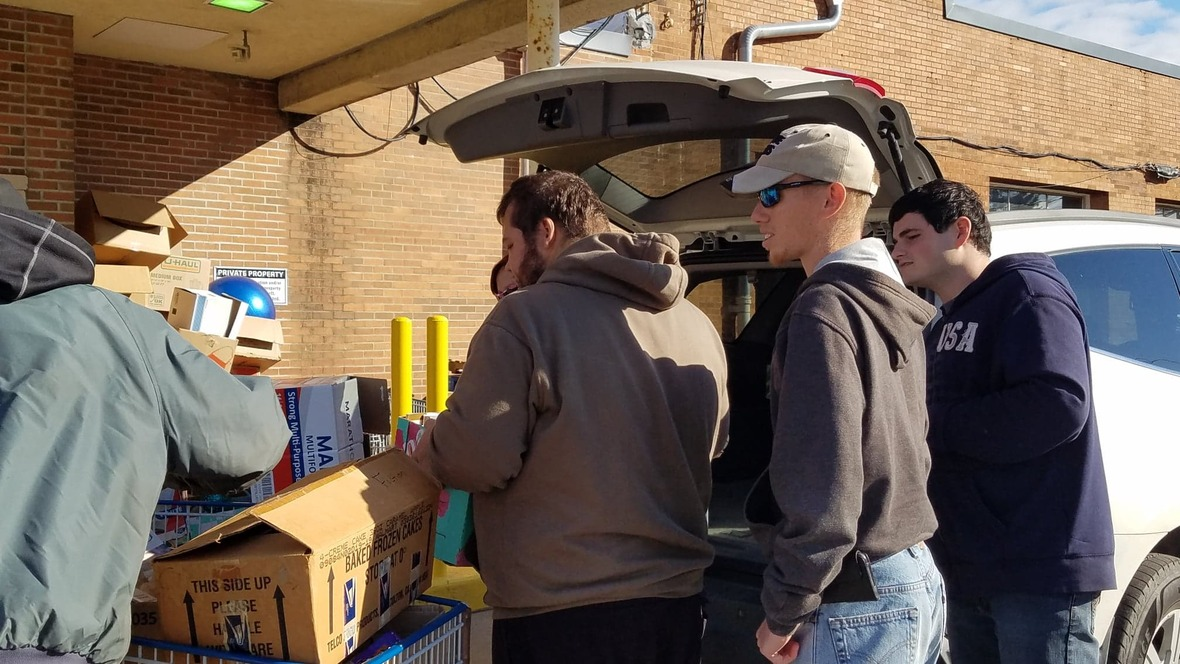Goodwill Drop-off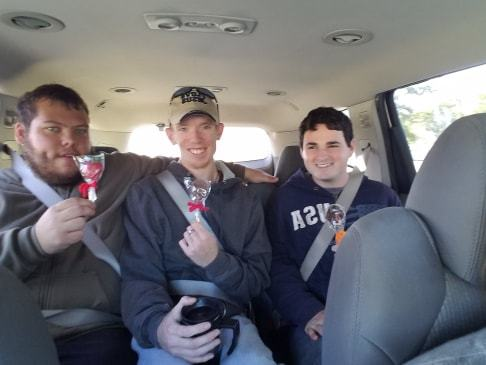Chocolate!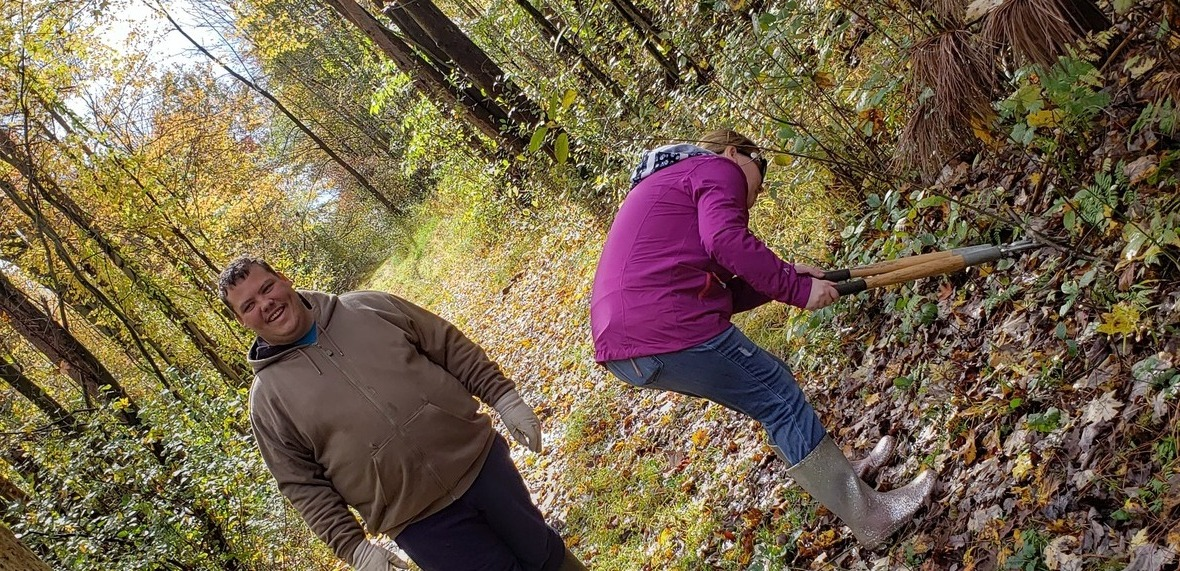Time in the Woods
 /* styles */ We wrapped up last week with a trip to Boardman. We dropped off the last of the books and knick-knacks at Goodwill, grabbed some supplies at Dollar General, then headed back to North Lima. We celebrated our hard work by enjoying chocolate on a stick from Rhodes.

# Friendsgiving 2019

 table.module-28{width:0%;padding:0}table div table+table+table+table+table+table+table+table+table+table+table+table+table+table+table+table+table+table+table+table+table+table+table+table+table+table+table+table+table div table{width:0%;float:none;margin-left:auto;margin-right:auto;padding:0}table div table+table+table+table+table+table+table+table+table+table+table+table+table+table+table+table+table+table+table+table+table+table+table+table+table+table+table+table+table div table a{border:0 none;text-decoration:none}table div table+table+table+table+table+table+table+table+table+table+table+table+table+table+table+table+table+table+table+table+table+table+table+table+table+table+table+table+table div table img{width:100%!important;border:0 none;text-decoration:none}table div table+table+table+table+table+table+table+table+table+table+table+table+table+table+table+table+table+table+table+table+table+table+table+table+table+table+table+table+table div table td{width:100%;padding:0}/* styles */
 table div table+table+table+table+table+table+table+table+table+table+table+table+table+table+table+table+table+table+table+table+table+table+table+table+table+table+table+table+table+table+table div table{width:100%;padding:0}table div table+table+table+table+table+table+table+table+table+table+table+table+table+table+table+table+table+table+table+table+table+table+table+table+table+table+table+table+table+table+table div table img{width:96.23%;padding:0;float:none}table div table+table+table+table+table+table+table+table+table+table+table+table+table+table+table+table+table+table+table+table+table+table+table+table+table+table+table+table+table+table+table div table td{width:100%;padding:0 1.88% 18px}/* styles */# OUTREACH and MISSION OPPORTUNITIES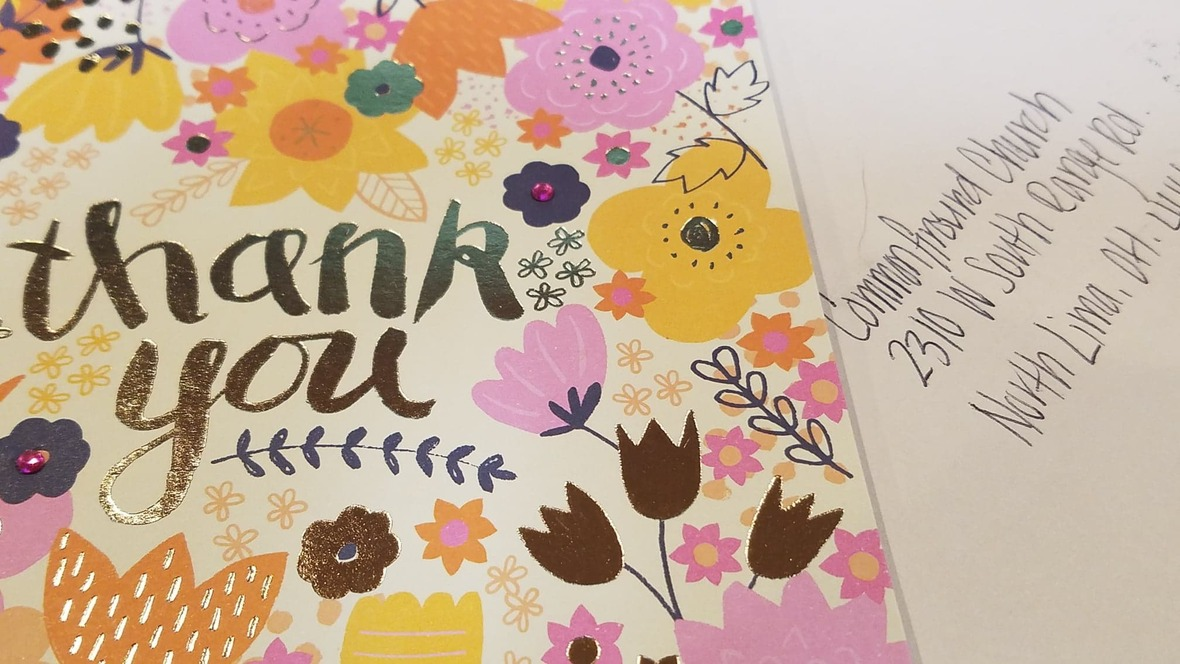## Beautiful Thank You Note

Read the thank you card that is on the front desk to see a snapshot of how our Giveaway impacts the community. We appreciate this writer enormously and we pray that this Giveaway can continue to benefit people for years to come.

## Ongoing Outreach/Mission Opportunity

Coat & Clothing & More Drive
Keep saving all your clothing and outerwear items for next year's giveaway. Tell your friends, family, neighbors, and co-workers that we will accept donations all year! Clothes for all ages and sizes needed, along with coats, bags, purses, shoes, housewares, books, toys...whatever you think might help someone in need. Drop items off in the collection barrel or take them up to the Green Room.

 /* styles */ ~~~~~~~
 /* styles */ For I was hungry and you gave me food; I was thirsty and you gave me a drink; I was a stranger and you took me in; I was naked and you clothed me; I was sick and you visited me. I was in prison and you came to me. .....When you did it to one of the least of these my brothers and sisters, you were doing it to me. Matthew 25: 35-36, 40 ~ What can YOU do?
 table div table+table+table+table+table+table+table+table+table+table+table+table+table+table+table+table+table+table+table+table+table+table+table+table+table+table+table+table+table+table+table+table+table+table+table+table+table div table{width:100%;padding:0}table div table+table+table+table+table+table+table+table+table+table+table+table+table+table+table+table+table+table+table+table+table+table+table+table+table+table+table+table+table+table+table+table+table+table+table+table+table div table img{width:96.23%;padding:0;float:none}table div table+table+table+table+table+table+table+table+table+table+table+table+table+table+table+table+table+table+table+table+table+table+table+table+table+table+table+table+table+table+table+table+table+table+table+table+table div table td{width:100%;padding:0 1.88% 18px}/* styles */# LOCAL COMMUNITY NEWS/EVENTS

 /* styles */ If you know of any interesting news or events, please let us know, so that we can pass it on to others!

# Crown Theater Production's Special Needs Show

Saturday & Sunday at 2pm
Don't miss Dustin, Tori, and many more of our friends on the stage! Sure to be a great time!
Break a leg, Dustin & Tori. We are so proud of you!

 table.module-40{width:0%;padding:0}table div table+table+table+table+table+table+table+table+table+table+table+table+table+table+table+table+table+table+table+table+table+table+table+table+table+table+table+table+table+table+table+table+table+table+table+table+table+table+table+table+table div table{width:0%;float:none;margin-left:auto;margin-right:auto;padding:0}table div table+table+table+table+table+table+table+table+table+table+table+table+table+table+table+table+table+table+table+table+table+table+table+table+table+table+table+table+table+table+table+table+table+table+table+table+table+table+table+table+table div table a{border:0 none;text-decoration:none}table div table+table+table+table+table+table+table+table+table+table+table+table+table+table+table+table+table+table+table+table+table+table+table+table+table+table+table+table+table+table+table+table+table+table+table+table+table+table+table+table+table div table img{width:100%!important;border:0 none;text-decoration:none}table div table+table+table+table+table+table+table+table+table+table+table+table+table+table+table+table+table+table+table+table+table+table+table+table+table+table+table+table+table+table+table+table+table+table+table+table+table+table+table+table+table div table td{width:100%;padding:0}/* styles */

# Fall Fun

Great Pumpkin Party

Town Center at Firestone Farms
October 26, 5-10pm
Trick or Treating for kids at the shops. Halloween/fall themed games for the kids along with the regular games at the Clock Tower. On the big screens, football, and maybe a special Halloween themed movie. Free event, held rain or shine.

 /* styles */ Colors and Reflections Mill Creek Park's Fellows Riverside Gardens October 27, 2-4pm Join Naturalist Marilyn Williams for a hike above and alongside Lake Glacier to enjoy the colors of autumn and their reflections upon the water. Call Fellows Riverside Gardens, 330.740.7116, for details. Moderate, 3 mi.
 table div table+table+table+table+table+table+table+table+table+table+table+table+table+table+table+table+table+table+table+table+table+table+table+table+table+table+table+table+table+table+table+table+table+table+table+table+table+table+table+table+table+table+table+table div table{width:100%;padding:0}table div table+table+table+table+table+table+table+table+table+table+table+table+table+table+table+table+table+table+table+table+table+table+table+table+table+table+table+table+table+table+table+table+table+table+table+table+table+table+table+table+table+table+table+table div table img{width:96.23%;padding:0;float:none}table div table+table+table+table+table+table+table+table+table+table+table+table+table+table+table+table+table+table+table+table+table+table+table+table+table+table+table+table+table+table+table+table+table+table+table+table+table+table+table+table+table+table+table+table div table td{width:100%;padding:0 1.88% 18px}/* styles */# CELEBRATE!

October 25 - National Breadstick Day
October 26 - Barry & Jen Lynch
October 27 - National Black Cat Day
October 28 - Meagan Tehua
October 29 - National Oatmeal Day

 table.module-45{width:0%;padding:0}table div table+table+table+table+table+table+table+table+table+table+table+table+table+table+table+table+table+table+table+table+table+table+table+table+table+table+table+table+table+table+table+table+table+table+table+table+table+table+table+table+table+table+table+table+table+table div table{width:0%;float:none;margin-left:auto;margin-right:auto;padding:0}table div table+table+table+table+table+table+table+table+table+table+table+table+table+table+table+table+table+table+table+table+table+table+table+table+table+table+table+table+table+table+table+table+table+table+table+table+table+table+table+table+table+table+table+table+table+table div table a{border:0 none;text-decoration:none}table div table+table+table+table+table+table+table+table+table+table+table+table+table+table+table+table+table+table+table+table+table+table+table+table+table+table+table+table+table+table+table+table+table+table+table+table+table+table+table+table+table+table+table+table+table+table div table img{width:100%!important;border:0 none;text-decoration:none}table div table+table+table+table+table+table+table+table+table+table+table+table+table+table+table+table+table+table+table+table+table+table+table+table+table+table+table+table+table+table+table+table+table+table+table+table+table+table+table+table+table+table+table+table+table+table div table td{width:100%;padding:0}/* styles */

# ON THE CG CALENDAR

October 24-30 . . .
Today - Transition Class
Sunday, 11am - Worship
Sunday, 6pm - YG
Wednesday, 9:30am - Ladies Bible Study
Wednesday - Mohawk Class
Wednesday, 6pm - Session Meeting/* styles */ Seeking to reflect the unconditional love of God, our mission is to help people connect - to God, to one another, and to God's purpose for the world.
 table div table+table+table+table+table+table+table+table+table+table+table+table+table+table+table+table+table+table+table+table+table+table+table+table+table+table+table+table+table+table+table+table+table+table+table+table+table+table+table+table+table+table+table+table+table+table+table+table+table+table div table{width:100%;padding:0}table div table+table+table+table+table+table+table+table+table+table+table+table+table+table+table+table+table+table+table+table+table+table+table+table+table+table+table+table+table+table+table+table+table+table+table+table+table+table+table+table+table+table+table+table+table+table+table+table+table+table div table img{width:96.23%;padding:0;float:none}table div table+table+table+table+table+table+table+table+table+table+table+table+table+table+table+table+table+table+table+table+table+table+table+table+table+table+table+table+table+table+table+table+table+table+table+table+table+table+table+table+table+table+table+table+table+table+table+table+table+table div table td{width:100%;padding:0 1.88% 18px}/* styles *//* styles */ Come grow with us! Service Time: Sunday @ 11:00am Common Ground Church Community 2310 West South Range Road North Lima, Ohio 44452 ~~~~~~~~~~~~~~~~~~~~~~~~~~~~~~~~~~~~~~~~~~~~ For more information, call 330-549-9408 or contact us by email at commonground.secretary@gmail.com.
 /* styles */